# Structured reference in Excel tables

This tutorial explains the basics of Excel structured references and shares some tricks on using them in real-life formulas.

One of the most useful features of Excel tables are structured references. When you have just stumbled upon a special syntax for referencing tables, it may look boring and confusing, but after experimenting a bit you will surely see how useful and cool this feature is.

## Excel structured reference

A structured reference, or table reference, is special way for referencing tables and their parts that uses a combination of table and column names instead of cell addresses.

This special syntax is required because Excel tables (vs. ranges) are very powerful and resilient, and normal cell references cannot adjust dynamically as data is added or removed from a table.

For example, to sum the values in cells B2:B5, you use the SUM function with a usual range reference:

`=SUM(B2:B5)`

To add up the numbers in the "Sales" column of Table1, you use a structured reference:

`=SUM(Table1[Sales])`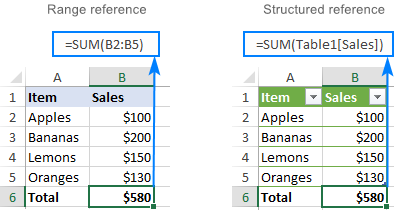### Key features of structured references

Compared to standard cell references, table references have a number of advanced features.

#### Easily created

To add structured references to your formula, you simply select the table cells you want to refer to. The knowledge of a special syntax is not required.

#### Resilient and automatically updated

When you rename a column, references are automatically updated with the new name, and a formula does not break. Moreover, as you add new rows to the table, they are immediately included in the existing references, and the formulas calculate the full set of data.

So, whatever manipulations you do with your Excel tables, you don't have to worry about updating the structured references.

#### Can be used inside and outside a table

Structured references can be used in formulas both inside and outside an Excel table, which makes locating tables in large workbooks easier.

#### Formula auto-fill (calculated columns)

To perform the same calculation in each table row, it is enough to enter a formula in just one cell. All other cells in that column are filled automatically.

## How to create a structured reference in Excel

Making a structured reference in Excel is very easy and intuitive.

If you are working with a range, convert it to an Excel table first. For this, select all the data and press Ctrl + T. For more information, please see How to create a table in Excel.

To create a structured reference, this is what you need to do:

1. Start typing a formula as usual, beginning with the equality sign (=).
2. When it comes to the first reference, select the corresponding cell or range of cells in your table. Excel will pick up the column name(s) and create an appropriate structured reference for you automatically.
3. Type the closing parenthesis and press Enter. If the formula is created inside the table, Excel automatically fills the entire column with the same formula.

As an example, let's add up the sales numbers for 3 months in each row of our sample table, named Sales. For this, we type =SUM( in E2, select B2:D2, type the closing parenthesis, and press Enter: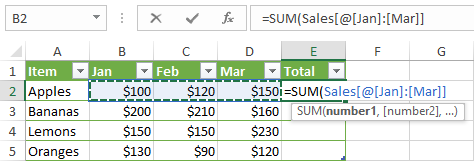As the result, the whole column E is auto-filled with this formula:

`=SUM(Sales[@[Jan]:[Mar]])`

Though the formula is the same, the data is calculated in each row individually. To understand the inner mechanics, please take a look at the table reference syntax.

If you are entering a formula outside the table, and that formula requires only a range of cells, a faster way to make a structured reference is this:

1. After the opening parenthesis, start typing the table name. As you type the first letter, Excel will show all matching names. If necessary, type a couple more letters to narrow down the list.
2. Use the arrow keys to select the table name in the list.
3. Double-click the selected name or press the Tab key to add it to your formula.
4. Type the closing parenthesis and press Enter.

For example, to find the largest number in our sample table, we start typing the MAX formula, after the opening parenthesis type "s", select the Sales table in the list, and press Tab or double-click the name.

As the result, we have this formula:

`=MAX(Sales)`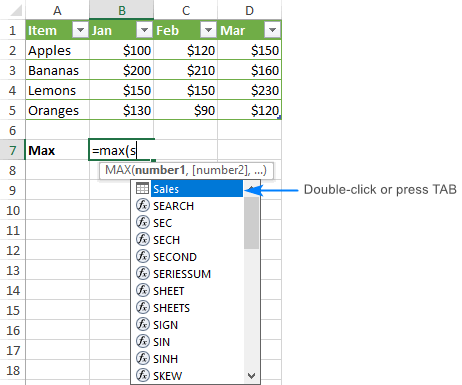## Structured reference syntax

As already mentioned, you do not need to know the syntax of structured references to include them in your formulas, however it will help you understand what each formula is actually doing.

Usually, a structured reference is represented by a string that begins with a table name and ends with a column specifier.

As an example, let's break down the following formula that adds up the totals of the South and North columns in the table named Regions: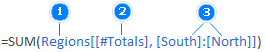The reference includes three components:

1. Table name
2. Item specifier
3. Column specifiers

To see what cells are actually calculated, select the formula cell and click anywhere in the formula bar. Excel will highlight the referenced table cells: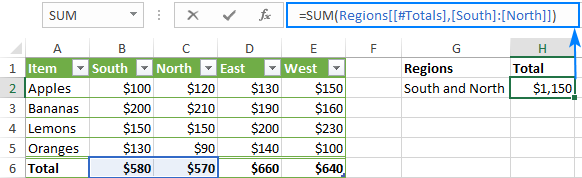### Table name

The table name references only the table data, without header row or total rows. It can be a default table name like Table1 or a custom name like Regions. To give a custom name to your table, carry out these steps.

If your formula is located within the table it refers to, the table name is usually omitted because it is implied.

### Column specifier

Column specifier references the data in the corresponding column, without the header row and total row. A column specifier is represented by the column name enclosed in brackets, e.g. [South].

To refer to more than one contiguous columns, use the range operator like [[South]:[East]].

### Item specifier

To refer to specific parts of a table, you can use any of the following specifiers.

 Item specifier Refers to [#All] The entire table, including table data, column headers and total row. [#Data] The data rows. [#Headers] The header row (column headers). [#Totals] The total row. If there's no total row, it returns null. [@Column_Name] The current row, i.e. the same row as the formula.

Please notice that the pound sign (#) is used with all the item specifiers, except the current row. To refer to the cells in the same row where you enter the formula, Excel uses the @ character followed by the column name.

For example, to add numbers in the South and West columns of the current row, you'd use this formula:

`=SUM(Regions[@South], Regions[@West])`

### Structured reference operators

The following operators allow you to combine different specifiers and add even more flexibility to your structured references.

#### Range operator (colon)

As with normal range references, you use a colon (:) to refer to two or more adjacent columns in a table.

For example, the below formula adds up the numbers in all the columns between South and East.

`=SUM(Regions[[South]:[East]])`

#### Union operator (comma)

To refer to non-adjacent columns, separate the column specifiers with commas.

For instance, here's how you can sum the data rows in the South and West columns.

`=SUM(Regions[South], Regions[West])`

#### Intersection operator (space)

It is used to refer to a cell at the intersection of a specific row and column.

For example, to return a value at the intersection of the Total row and West column, use this reference:

`=Regions[#Totals] Regions[[#All],[West]]`

Please notice that the [#All] specifier is required in this case because the column specifier does not include the total row. Without it, the formula would return #NULL!.

### Table reference syntax rules

#### 1. Enclose specifiers in brackets

All column and special item specifiers must be enclosed in [square brackets].

A specifier that contains other specifiers should be wrapped in outer brackets. For example, Regions[[South]:[East]].

#### 2. Separate inner specifiers with commas

If a specifier contains two or more inner specifiers, those inner specifiers need to be separated with commas.

For example, to return the header of the South column, you type a comma in between [#Headers] and [South] and enclose this whole construction in an additional set of brackets:

`=Regions[[#Headers],[South]]`

#### 3. Do not use quotation marks around column headers

In table references, column headers don't require quotes whether they are text, numbers or dates.

#### 4. Use a single quotation mark for some special characters in column headers

In structured references, some characters such as left and right brackets, pound sign (#) and single quotation mark (') have special meaning. If any of the above characters is included in a column header, a single quotation mark needs to be used before that character in a column specifier.

For example, for the column header "Item #", the specifier is [Item '#].

#### 5. Use spaces to make structured references more readable

To improve the readability of your table references, you can insert spaces in-between specifiers. Normally, it is considered a good practice to use spaces after commas. For example:

`=AVERAGE(Regions[South], Regions[West], Regions[North])`

## Excel table references - formula examples

To gain more understanding about structured references in Excel, let's go over a few more formula examples. We'll try to keep them simple, meaningful and useful.

### Find the number of rows and columns in an Excel table

To get the total columns and rows count, use the COLUMNS and ROWS functions, which only require the table name:

COLUMNS(table)
ROWS(table)

For example, to find the number of columns and data rows in the table named Sales, use these formulas:

`=COLUMNS(Sales)`

`=ROWS(Sales)`

To include the header and total rows in the count, use the [#ALL] specifier:

`=ROWS(Sales[#All])`

The below screenshot shows all the formulas in action: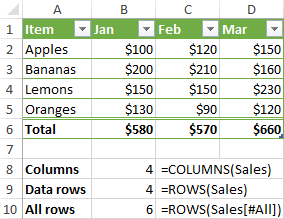### Count blanks and non-blanks in a column

When counting something in a specific column, be sure to output the result outside the table, otherwise you might end up with circular references and wrong results.

To count blanks in a column:

COUNTBLANK(table[column])

To count non-blank cells in a column:

COUNTA(table[column])

For example, to find out how many cells in the Jan column are empty and how many contain data, use these formulas:

Blanks: `=COUNTBLANK(Sales[Jan])`

Non-blanks: `=COUNTA(Sales[Jan])`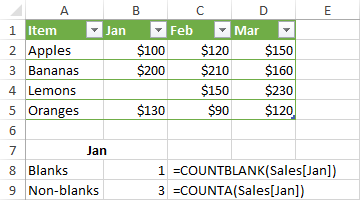To count non-blank cells in visible rows in a filtered table, use the SUBTOTAL function with function_num set to 103:

`=SUBTOTAL(103,Sales[Jan])`

### Sum in an Excel table

The fastest way to add up numbers in an Excel table is to enable the Total Row option. To do this, right click any cell within the table, point to Table, and click Totals Row. The total row will appear at the end of your table straight away.

Sometimes Excel may assume you want to total only the last column and leaves other cells in the Total row blank. To fix this, select an empty cell in the Total row, click the arrow that appears next to the cell, and then select the SUM function in the list: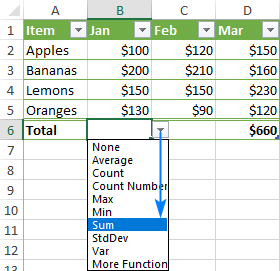This will insert a SUBTOTAL formula that sums values only in visible rows, ignoring filtered-out rows:

`=SUBTOTAL(109,[Jan])`

Please note that this formula works only in the Total row. If you try to manually insert it in a data row, this would create a circular reference and return 0 as the result. A SUM formula with a structured reference won't work either for the same reason: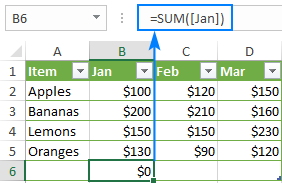So, if you want the totals inside the table, you need to either enable the Total row or use a normal range reference such as:

`=SUM(B2:B5)`

Outside the table, the SUM formula with a structured reference works just fine:

`=SUM(Sales[Jan])`

Please note that unlike SUBTOTAL, the SUM function adds up values in all the rows, visible and hidden.

## Relative and absolute structured references in Excel

By default, Excel structured references behave in the following way:

• Multiple column references are absolute and do not change when formulas are copied.
• Single column references are relative and change when dragged across columns. When copied/pasted via a corresponding command or shortcuts (Ctrl+C and Ctrl+V), they do not change.

In situations when you need a combination of relative and absolute table references, there is no way to copy the formula and keep table references correct. Dragging the formula will change the references to single columns, and copy/pasting shortcuts will make all the references static. But there are a couple of simple tricks to get around!

### Absolute structured reference to single column

To make a single column reference absolute, repeat the column name to formally turn it into a range reference.

#### Relative column reference (default)

`table[column]`

#### Absolute column reference

`table[[column]:[column]]`

To make an absolute reference for the current row, prefix the column identifier by the @ symbol:

`table[@[column]:[column]]`

To see how relative and absolute table references work in practice, please consider the following example.

Supposing you want to add up the sales numbers for a specific product for 3 months. For this, we enter the target product name in some cell (F2 in our case) and use the SUMIF function to get the total of Jan sales:

`=SUMIF(Sales[Item], \$F\$2, Sales[Jan])`

The problem is that when we drag the formula to the right to calculate the totals for the other two months, the [Item] reference changes, and the formula breaks: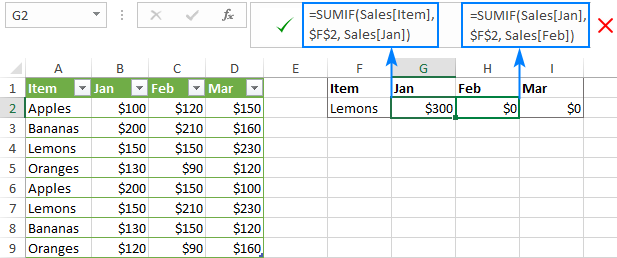To fix this, make the [Item] reference absolute, but keep [Jan] relative:

`=SUMIF(Sales[[Item]:[Item]], \$F\$2, Sales[Jan])`

Now, you can drag the modified formula to other columns and it works perfectly: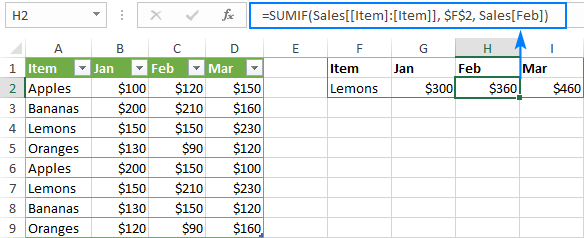### Relative structured reference to multiple columns

In Excel tables, structured references to several columns are absolute by their nature and remain unchanged when copied to other cells.

To me, this behavior is very reasonable. But if you need to make a structured range reference relative, prefix each column specifier with the table name and remove the outer square brackets as shown below.

#### Absolute range reference (default)

`table[[column1]:[column2]]`

#### Relative range reference

`table[column1]:table[column2]`

To refer to the current row inside the table, use the @ symbol:

`[@column1]:[@column2]`

For example, the below formula with an absolute structured reference adds up the numbers in the current row of the Jan and Feb columns. When copied to another column, it will still sum Jan and Feb.

`=SUM(Sales[@[Jan]:[Feb]])`

In case you want the reference to change based on a relative position of the column where the formula is copied, make it relative:

`=SUM(Sales[@Jan]:Sales[@Feb])`

Please notice the formula transformation in column F (the table name is omitted because the formula is inside the table):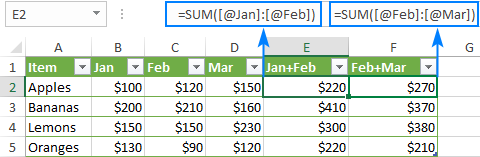That's how you make table references in Excel. To have a closer look at the examples discussed in this tutorial, feel free to download our sample workbook to Excel Structured Reference. I thank you for reading and hope to see you on our blog next week.

### 12 Responses to "Structured reference in Excel tables"

1.Dave Shorr says:

I've discovered structured references and want to do more with them. Seems like they only work as intended (auto filling columns) on the same worksheet with the table / query. Is there a trick to doing this from a different worksheet?

•Svetlana Cheusheva (Ablebits.com Team) says:

Hi Dave,
Structured references can also be used from a different worksheet. When making a formula, you just need to select cells in the original table using the mouse. In this tutorial, you can find some examples of formula with structured references outside a table.

2.Shane says:

Hi
I'm trying to calulate the sequential duplicate number in a list of Id Numbers in a table. There are different numbers of duplicates for each Id number. Because the table is downloaded as an excel template from Dynamics I need to use the structured reference (using cell references doesn't calculate in the downloaded spreadsheet as the formula doesn't copy down without structured references). Normally I would just use =countif(d\$2:d2,d2), but this doesn't automatically calculate when downloaded from Dynamics. When I create my version of this in a structured reference formula it doesn't work. I've tried =COUNTIF([Form '#]:[@[Form '#]],[@[Form '# ]]) where Form # is the field in the table but it proviudes me with the number of duplicate records in every row. I've tried a few alternate versions but they don't work either. Thanks in advance, Shane

•Matt Tarler says:

Shane, I was looking something else up and saw your comment. Sorry I didn't see it earlier. If you are still wondering the problem is that the first [Form '#] of your "range" is already the entire column and hence why all results are total number of that duplicates. Try [[#Headers],[Form '#]] instead to force it to use the header cell of that column for your range. So the new formula would be:

3.Sergio says:

I see your examples on structured references in columns. How can I use this in rows? I want a cumulative total column next to a column of values. I can do this with =SUM(\$B\$3:B6) in column C but can I do this with structured references? My table has a column called DESCRIPTION and one called AMOUNT. I would like a cumulative column with a running total.

Thanks for the help
Sergio

•Matt Tarler says:

Hi Sergio, similar to my answer above you can use the Header to 'fix' the top. Try:

4.Derrick says:

I'm having an issue. I'm using Table structured references in my linear regression sheets. However, after I have everything setup like I want it and I save and re-open the file, the structured reference formulas turn into regular formulas referencing the rows/columns of the current data in the sheet like a regular formula. I need my structured references to stay intact so they will grow and shrink as I add and remove data to the tables.
for example:
=MEDIAN((Table14[CostPerUnit]))
after saving and re-opening turns into:
=MEDIAN('Sheet1'!\$K\$16:\$K\$69)

Normal behavior? Anyway to avoid it or am I missing something? Any help is appreciated!! Thank you!

•G JONES says:

I have the same problem. Any solution?

5.nooma says:

awesome tutorial! I'm currently diving into courses of excel provided by pwc which lacks the explanation of this. Nice job!

6.Maria says:

Hi,
If the name of the column i want to use is in a specific cell, how is the correct notacion of this reference.
Example
In cell B1 = Amount_20
Instead of writing the formula "=ProfitAndLossStandard__3[@[Amount_20]]" I tried to use "=ProfitAndLossStandard__3[@[B1]]" but did not work.
How do I make this reference correctly?

7.Sergio says:

Matt, thank you for your help. I was able to make it work. I did need to include the table name, but that may be my version of Excel

Formula, (in a column titled 'Total'), that worked for me:
8.Chris says: